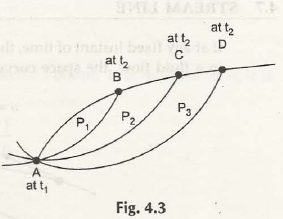A line is drawn and marked following fluid particles show the streak line. It concentrates on every single particle to highlight the streak line of each and every particle as shown in Fig. 4.3Let us consider a fixed point A at time t1 is in the fluid flow. Three particles P1, P2 and P3 follows unsteady flow and traced new paths after crossing A to reach B, C and D respectively at time t2. Now, draw line join AB, AC and AD to make streak lines.

From Lagrangian method, if the fluid particle passes through any fixed point is given by,

= S (0 , t)

So, the equation of streak line can be written as-

At time t,

= f (0 , t)

Or,

=f[F(1 , t), t]

It shows the equation for streak line w.r.t fixed point.

Links of Previous Main Topic:-

Links of Next Mechanical Engineering Topics:-

### Submit Your Assignment### Customer Reviews

My Homework Help
Rated 5.0 out of 5 based on 510 customer reviews at